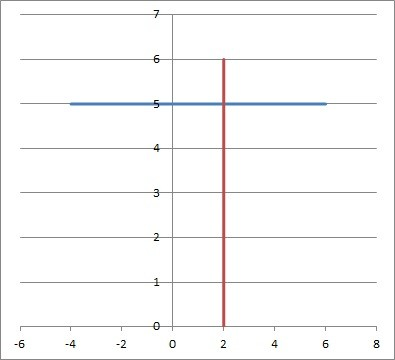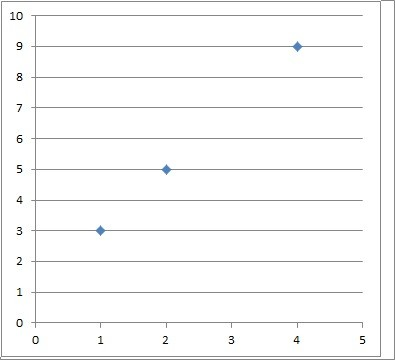# What Is An Ordered Pair? (6 Common Questions Answered)

Ordered pairs are used in math to define relations and functions.  They are also used when graphing these relations and functions.

So, what is an ordered pair?  An ordered pair is two items (usually numbers) that are written in parentheses and separated by a comma. The order of the items matters, so the ordered pair (1, 3) is not the same as the ordered pair (3, 1). Often, the first item in the pair is an input, and the second item in the pair is an output.

Of course, ordered pairs help us to graph in 2 dimensions, but we can also have ordered triples (3 dimensions) and ordered n-tuples (in n dimensions).

Let’s get started.

## What Is An Ordered Pair?

An ordered pair (a, b) is a pair of two items: the first is a, and the second is b.  The items a and b are often numbers, but they could be anything, including:

• Letters
• Words
• Sets of Numbers

The order of the items matters, so that the ordered pair (a, b) is not always the same as (b, a) (except in the special case when a = b).

For example, the ordered pair (2, 5) is not the same as the ordered pair (5, 2).  For two ordered pairs (a, b) and (c, d) to be the same, we need both a = c and b = d.

Generally, we think of the first item in an ordered pair as an input (independent variable) and the second item in an ordered pair as an output (dependent variable).

This is helpful when we talk about relations or functions, which connect an input variable and an output variable (often for the purpose of graphing).

### Can Ordered Pairs Be A Function?

A set of ordered pairs can be a function, but only in a special case (more on this below).

First, an ordered pair is a single pair of items, such as (1, 3).

A relation is a set of one or more ordered pairs.

For example, the set {(1, 3)} is a relation (this relation only has one ordered pair).

The set {(1, 3), (2, 5)} is also a relation (this relation has two ordered pairs).

The set {(1, 3), (2, 5), (3, 12)} is also a relation (this relation has three ordered pairs).

A function is a special type of relation: one where each input has only one output.  That is, if any two ordered pairs in the set have the same input (first item), then they have the same output (second item).

This means that a function avoids ambiguity of output.  For a given input, we know that there is only one possible output.

For example, the set {(1, 3), (2, 5)} is a function (each input has only one output: 1 has an output of 3, and 2 has an output of 5).

The set {(1, 3), (1, 4)} is not a function, since an input of 1 has two possible outputs: 3 and 4.  If we were to graph the ordered pairs in this set, the vertical line x = 1 would pass through the set twice (you can learn more about the vertical line test here).

The set {(1, 3), (2, 3)} is a function (each input has only one output: 1 has an output of 3, and 2 has an output of 3 – the fact that two different inputs have the same output means the function is not one-to-one).

#### Can One Ordered Pair Be A Function?

One ordered pair can be a function.  In fact, a set of one ordered pair is always a function (and thus always a relation).

The reason is that there is only one input and one output.  The set with one ordered pair {(a, b)} is a relation with only one input, which is a.

The only possible output is b, and so the relation is a function.  Another way to think of it is that any vertical line will pass through the graph of this relation at most once.

### Can An Ordered Pair Have Three Numbers?

An ordered pair cannot have three numbers.  An ordered pair is defined to be two numbers (or items).

However, an ordered triple does have three numbers.  An ordered triple is a set of three items (a, b, c) where the order of the items matters.

The only way that two ordered triples (a, b, c) and (d, e, f) are the same is if a = d, b = e, and c = f.

For example, all of the following ordered triples are different:

• (1, 2, 3)
• (1, 3, 2)
• (2, 1, 3)
• (2, 3, 1)
• (3, 1, 2)
• (3, 2, 1)

So for any set of three distinct items a, b, and c, there are 3! = 6 possible ordered triples (3 choices for the first item, 2 choices for the second item, and 1 choice for the third item: 3*2*1 = 3!).

Note that these ordered triples would be graphed in 3-dimensional space.

We can also have 4, 5, or more ordered items.  An ordered n-tuple has n items, and the order matters (as it does for ordered pairs and ordered triples).

For n distinct items x1, x2, … , xn, there are n! possible ordered n-tuples (n choices for the first item, n – 1 choices for the second item, … 1 choice for the nth item: n*(n – 1)*…*2*1 = n!).

### Can An Ordered Pair Have A Fraction?

An ordered pair can have a fraction, decimal, or radical as one of the items.  For example, the following are all valid ordered pairs:

• (1/5, 6)
• (5.7, 2/3)
• (7/2, 6/5)
• (√7, 3)

The exception is if we restrict the items to a particular set of numbers.

For example, if we restrict the items in an ordered pair to rational numbers, then there can be no radicals.  In that case, (√7, 3) would not be a valid ordered pair.

If we restrict the items in an ordered pair to integers, then there can be no fractions or decimals.  In that case, (1/5, 6), (5.7, 2/3), and (7/2, 6/5) would not be valid ordered pairs.

### How To Graph An Ordered Pair

To graph an ordered pair (a, b), you can think of it as finding the intersection between two lines:

• The vertical line x = a
• The horizontal line y = b

Since a vertical line and a horizontal line are perpendicular, they will intersect at exactly one point: (a, b), which corresponds to this ordered pair on a graph in 2-dimensional space (a coordinate plane).

#### Example: How To Graph An Ordered Pair

Let’s say we want to graph the ordered pair (2, 5).

Then we would find the intersection of two lines:

• The vertical line x = 2
• The horizontal line y = 5

You can see these two lines graphed in the picture below, along with their intersection point at (2, 5):We can see that the vertical line x = 2 (red) and the horizontal line y = 5 (blue) intersect at the point (2, 5).

Once you get the hang of it, you don’t need to draw the lines – you can just figure out where they intersect and plot the single point.

### How To Graph A Relation

To graph a relation, we simply graph the entire set of all ordered pairs in the relation.

#### Example 1: How To Graph A Relation (Discrete)

Let’s say we want to graph the relation {(1, 3), (2, 5), (4, 9)}.

Then we graph each of the three ordered pairs, as shown in the picture below:This graph shows the three ordered pairs (1, 3) (bottom left point), (2, 5) (middle point), and (4, 9) (top right point).

Note that this relation is a function, since it passes the vertical line test.  Any vertical line will intersect the graph of the relation at most once.

#### Example 2: How To Graph A Relation (Continuous)

Let’s say we want to graph the relation {(x, y): y = 2x}

Then we graph all of the three ordered pairs in the relation.  Since there are infinitely many points, we will graph a couple of points and connect them.

• For x = 0, we get y = 2x = 2*0 = 0, so the ordered pair (0, 0) is on the graph.
• For x = 1, we get y = 2x = 2*1 = 2, so the ordered pair (1, 2) is on the graph.

We know that this relation will look like a line (since the equation is y = 2x, which has the slope-intercept form for a line: y = mx + b with m = 2 and b = 0).

You can see our two points and the relation graphed in the picture below:

Note that this relation is a function, since it passes the vertical line test.  Any vertical line will intersect the graph of the relation at most once.

This function is one-to-one, since it passes the horizontal line test.  Any horizontal line will intersect the graph of the relation at most once.

## Conclusion

Now you know what an ordered pair is and what they are used for.  You also know the answers to some common questions about this important concept.